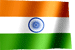RAMESH KRISHNA REDDYOnline Tutorials | PREV | TOP | NEXT

DRONA SERIES
COBOL STUDY MATERIAL

COBOL VERBS - SUBTRACT

 ``` COBOL VERBS - SUBTRACT SUBTRACT Verb Syntax 1 - SUBTRACT { literal-1 / data-item-1 } .. FROM data-item-2 .. Syntax 2 - SUBTRACT { literal-1 / data-item-1 } .. FROM { literal-2/data-item-2 } GIVING data-item-3 .. Rules - 1. All the values before the word FROM, summed up and subtracted from each data-item/literl after word FROM 2. If GIVING is used, the result will be stored in the data-items provided after the word GIVING. 3. If GIVING is not used, data-items provided after FROM will be used to store the results. Let us see some examples. Let us assume WS-A contains value 200 WS-B contains value 100 SUBTRACT 10 FROM WS-A. 10 will be subtracted from WS-A and the result will be stored in WS-A. i.e, after excution of above statement WS-A contains 190. SUBTRACT 10 FROM WS-A GIVING WS-B. 10 will be subtracted from WS-A and the result will be stored in WS-B. Please note there wont be any change in the value of WS-A. WS-A is used in the calcualtion, but the value in WS-A data item wont get changed. SUBTRACT 10 11 12 FROM WS-A GIVING WS-B. Numbes 10,11,12 will be summed up and subtracted from WS-A value , result will be stored in WS-B. No change to WS-A value. SUBTRACT 10 20 30 FROM WS-A WS-B WS-C. Numbers 10,20,30 will be summed up and the result value subtracted from WS-A, WS-B, WS-C and stored in the same data items. i.e., summed up value 10+20+30 = 60 , subtracted from WS-A, result will be stored in WS-A,60 subtracted from WS-B and the result will be stored in WS-B. 60 subtracted from WS-C and the result will be stored in WS-C. ```

NEXT CHAPTER TOPIC : COBOL

Visit COBOL books section in this site for good books